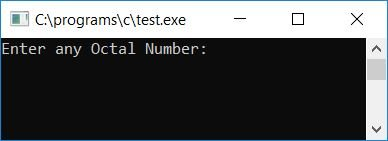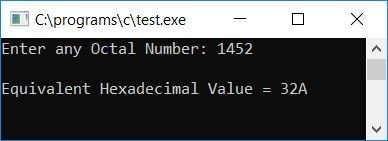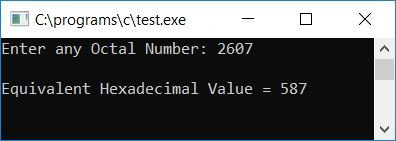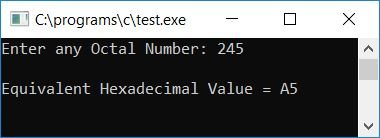# C Program to Convert Octal to Hexadecimal

In this article, we will learn how to create a program in C that converts any given octal number into its equivalent hexadecimal value. At last, we have also created a program using a user-defined function, OctToHex(), that does the same job.

But before going through the program, if you are not aware of

• Octal Number
• Octal to Hexadecimal Conversion Process

then refer to the octal to hexadecimal conversion step-by-step process. Now let's move on to the program.

## Octal to hexadecimal in C

To convert an octal number to a hexadecimal number in C programming, you have to ask the user to enter any octal number. Then convert it to hexadecimal and display the hex value as output. The question is, Write a program in C that converts any given octal number entered by the user (at run-time) to its equivalent hexadecimal value. The answer to this question is given below:

```#include<stdio.h>
#include<conio.h>
#include<string.h>
int main()
{
int octnum, rev=0, rem, count=0, hex=0, mul=1, i=0, k=0;
char binnum = "", hexnum;
printf("Enter any Octal Number: ");
scanf("%d", &octnum);
while(octnum!=0)
{
rem = octnum%10;
if(rem>7)
{
count++;
break;
}
rev = (rev*10) + rem;
octnum = octnum/10;
}
if(count==0)
{
octnum = rev;
while(octnum!=0)
{
rem = octnum%10;
switch(rem)
{
case 0: strcat(binnum, "000");
break;
case 1: strcat(binnum, "001");
break;
case 2: strcat(binnum, "010");
break;
case 3: strcat(binnum, "011");
break;
case 4: strcat(binnum, "100");
break;
case 5: strcat(binnum, "101");
break;
case 6: strcat(binnum, "110");
break;
case 7: strcat(binnum, "111");
break;
}
octnum = octnum/10;
}
while(binnum[k]!='\0')
k++;
count=1;
k--;
while(k>=0)
{
if(binnum[k]=='0')
rem = 0;
else
rem = 1;
hex = hex + (rem*mul);
if(count%4==0)
{
if(hex<10)
hexnum[i] = hex+48;
else
hexnum[i] = hex+55;
mul = 1;
hex = 0;
count = 1;
i++;
}
else
{
mul = mul*2;
count++;
}
k--;
}
if(count!=1)
hexnum[i] = hex+48;
if(count==1)
i--;
count = 0;
for(i=i; i>=0; i--)
{
if(hexnum[i]=='0' && count==0)
{
count++;
continue;
}
else
printf("%c", hexnum[i]);
}
}
else
printf("\nInvalid Octal Digit %d", rem);
getch();
return 0;
}```

As the above program was written in the Code::Blocks IDE, here is the first snapshot of the sample run:Now supply any octal number, say 1452, as input and press the ENTER key to see its hexadecimal equivalent as shown in the second snapshot of the sample run:Here is the final snapshot of another sample run:#### Program Explained

• Receive any octal number from the user at run-time, say 1452, as input.
• Now reverse the given octal number and initialize its reverse to the original number, say octnum itself.
• We reversed the number here using the modulus (%) operator, which returns the last digit every time we take modulus. For example, while applying the modulus operator in a way like 1452%10, we will get 2 as a result, and 2 is the last digit, but after reversing the number, it will become 2541. And then, after applying the modulus operator in the same way as 2541%10, we will get 1 as a result, and 1 is the first digit of the original number, say 1452.
• While reversing the number, we have checked whether any digit is greater than 7 or not. As in the octal number system, all the digits must be less than or equal to 7. But if any digit is greater than 7, then we have incremented a variable, say count (it is initialize with 0 at the start of the program), so that we can check after exiting from the while loop that it holds its original value (0) or not; if it holds, then we have to proceed to convert the number from octal to hexadecimal; otherwise, we have to print a message like "You have entered an invalid octal digit."
• Now if count holds its original value, then program flow goes inside the if block.
• And rev (the octal number's inverse), or 2541, is assigned to octnum.
• Then, to convert the octal number to binary, we used a while loop.
• The condition of the while loop is octnum! = 0 or 2541! = 0. evaluates to true, and program flow goes inside the loop.
• There, octnum%10 or 2541%10 or 1 gets initialize to rem.
• Using switch case, we have checked what value is present inside the variable rem.
• Whatever the value in rem, concatenate it to the binnum character array.
• In order to concatenate each octal digit's binary equivalent (in string form) into a binnum character array one at a time, we have used the strcat() function of the string.h library.
• After concatenation, octnum (10, 2541/10, or 254) gets initialize to octnum
• And the program flow goes back to the condition of the loop, where octnum!=0 or 254!=0 evaluates to true, and the program flow again goes inside the loop and does the same operation as described above until the value of octnum becomes 0.
• After exiting the while loop, we have a binnum character array that holds 001100101010.
• Here 001, 100, 101, and 010 are the binary equivalents of 1, 4, 5, and 2.
• Now that we've used the while loop to count the length of the binnum character array, we know how many characters are contained within it.
• The length of the array binnum is set to a variable, say k.
• The value of k gets decremented as indexing in the array starts with 0.
• Now we have the binary equivalent of each octal digit.
• It's time to make a 4-4 binary pair from the right side and convert it into its hexadecimal equivalent.
• To do this, we have to start from the last index of the binnum array.
• At the first run of the while loop, the condition k>=0 or 8>=0 evaluates to true, therefore program flow goes inside the loop.
• Inside the loop, we have checked what the character present at the current index of the array binnum is; if it is 0, then we initialize 0 to rem, otherwise we initialize 1 to rem.
• And do the same procedure as told in the binary to hexadecimal.
• After doing all the above steps, we only have to print each hexadecimal digit one by one.
• Here we have used an if statement inside the for loop in printing hexadecimal digits, so that any zero (0) present at the beginning of the hexadecimal number gets skipped.

Note: You can also approach the second option, which is octal to decimal and then  decimal to hexadecimal. Because the second option is a little easier to create and understand than the first one, Therefore, the first option is used here, which converts octal to binary and then binary to hexadecimal.

You can follow both articles (used for the second option) to create the program yourself. I'm sure that will be very easy for you after you understand the first option as created above.

### Octal to Hexadecimal in C using a User-Defined Function

Let's write the same program for the same purpose, but this time with user-defined functions. The question is: "Write a program in C that converts octal to hexadecimal using a user-defined function named OctToHex()." The answer to this question is:

```#include<stdio.h>
#include<conio.h>
#include<string.h>
void OctToHex(int oct);
static int count, i;
char hexnum;
int main()
{
int octnum;
printf("Enter any Octal Number: ");
scanf("%d", &octnum);
OctToHex(octnum);
if(count==0)
printf("\nYou've entered an invalid octal digit");
else
{
count = 0;
for(i=i; i>=0; i--)
{
if(hexnum[i]=='0' && count==0)
{
count++;
continue;
}
else
printf("%c", hexnum[i]);
}
}
getch();
return 0;
}
void OctToHex(int oct)
{
int rev=0, rem, hex=0, mul=1, k=0;
char binnum = "";
while(oct!=0)
{
rem = oct%10;
if(rem>7)
{
count++;
break;
}
rev = (rev*10) + rem;
oct = oct/10;
}
if(count==0)
{
oct = rev;
while(oct!=0)
{
rem = oct%10;
switch(rem)
{
case 0: strcat(binnum, "000");
break;
case 1: strcat(binnum, "001");
break;
case 2: strcat(binnum, "010");
break;
case 3: strcat(binnum, "011");
break;
case 4: strcat(binnum, "100");
break;
case 5: strcat(binnum, "101");
break;
case 6: strcat(binnum, "110");
break;
case 7: strcat(binnum, "111");
break;
}
oct = oct/10;
}
while(binnum[k]!='\0')
k++;
count=1;
k--;
while(k>=0)
{
if(binnum[k]=='0')
rem = 0;
else
rem = 1;
hex = hex + (rem*mul);
if(count%4==0)
{
if(hex<10)
hexnum[i] = hex+48;
else
hexnum[i] = hex+55;
mul = 1;
hex = 0;
count = 1;
i++;
}
else
{
mul = mul*2;
count++;
}
k--;
}
if(count!=1)
hexnum[i] = hex+48;
if(count==1)
i--;
}
else
count=0;
}```

Here is the final snapshot of the sample run:Here we have declared the variables i and count as static variables, so that they can hold or remember their previous values. We have not initialized both the static variables with 0, because by default, static variables get initialized with 0 as their initial value automatically. We have declared both static variables and a character array hexnum[] as global variables to make all three variables known to both the main() and OctToHex() functions.

#### The same program in different languages

C Quiz

« Previous Program Next Program »